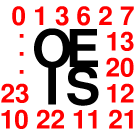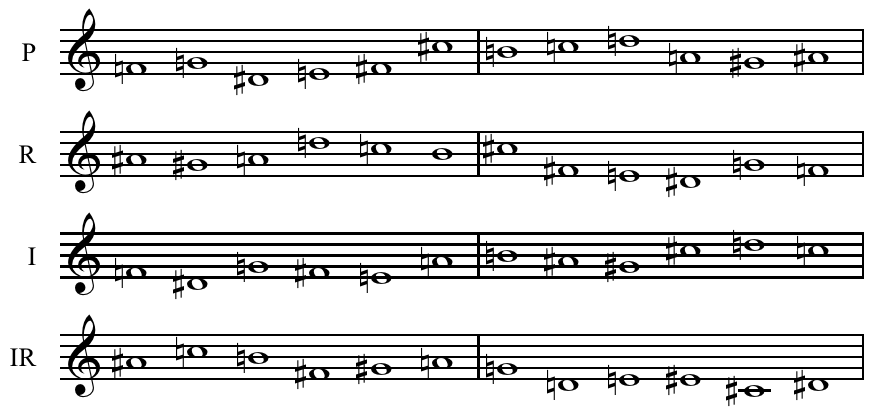# Blog » Music Composition & Math (Numbers & Sequences)

Reading Time: 8 minutesJuly 1, 2016

#### MUSIC COMPOSITION & MATH (NUMBERS/SEQUENCES)

May not music be described as the mathematics of the sense, mathematics as music of the reason?
The musician feels mathematics, the mathematician thinks music:
music the dream, mathematics the working life.

~ James Joseph Sylvester

Most people that have visited this blog before have come across various music and math related stuff already, like for example Music & GeometryIn this article I will share some interesting and fun-stuff about music composition and math. Let me start in this article with something I wrote in another article:

MUSIC = MATH
Music can be explained with math formulas.

How?

1. We use ratios – for example 2:1 (Octave) and 3:2 (Perfect 5th) – to “size-up” the intervals between 2 tones (more about that in the article “Music & Geometry” at Roel’s World).
2. A system of combined interval ratios generates a musical Temperament (Roel’s World).
3. With 3 or more tones a geometric relationship can be formed (Roel’s World article: “Music & Geometry“).
4. By using number sequences (consecutive intervals and/or patterns/cycles).

Temperaments and Tuning Systems, Geometry … all cool and so. But what about composing music with Mathematical sequences and patterns?

#### NUMBER SEQUENCES

Numbers and number sequences can be used in creative manners to compose music:
PI | PHI | FIBONACCI | NUMBER SEQUENCES & PROGRESSIONS | DODECAPHONY | NUMEROLOGY

PI (π)

The number π (Pi) is a mathematical constant, the ratio of a circle’s circumference to its diameter, commonly approximated as 3.14159… Being an irrational numberπ cannot be expressed exactly as a fraction (equivalently, its decimal representation never ends and never settles into a permanent repeating pattern). Here you can find the first one million digits

There are various musicians and composers that have experimented with using the digits of Pi as melody for their composition. Here’s one of the examples, a implementation by David Macdonald:

PHI (φ)

Next up is Phi (φ) = 1.61803398874989484820458683436563811772­… Phi is used as a symbol for the golden ratio and on other occasions in math and science.

Phi has been a source of inspiration for some musicians and composers. Here is an example by Michael Blake:

FIBONACCI SEQUENCE

The Fibonacci Sequence (0, 1, 1, 2, 3, 5, 8, 13, 21, 34, 55, 89, 144, 233, 377, 610, 987, …) is named after Italian mathematician Leonardo of Pisa, known as Fibonacci. His 1202 book Liber Abaci introduced the sequence to Western European mathematics, although the sequence had been described earlier as Virahanka numbers in Indian mathematics. Here you can find the first 1000 digits

In the example below Radomir Nowotarski has “related” the Fibonacci Sequence to the Lydian Mode (scale) and made the following assignment:

1=C, 2=D, 3=E, 4=F#, 5=G, 6=A, 7=B, 8=C, 9=D, 10=E, 11=F#, 12=G, 13=A et cetera. (see video).

1-8 are the intervals of the Scale (tonic-octave). The following numbers 9, 10, 11 (et cetera) represent intervals greater then the octave and have been added to the table to complete it with all possible intervals related to the scale used. Numbers like 4, 6, 7, 9, 10, 11, 12 (et cetera) are not part of the Fibonacci frequencies and haven’t been used for constructing the melody. This does not mean the tones related to those intervals are not used. “6=A” (not used) as well as “13=A“, as well as for example 34=A, 55=A, while 89=G (et cetera, see video).

This assignment can be applied with any other 7-tone scale (by replacing the 7 tones listed in the first column) or you could use the concept to build a table for a scale with a different number of tones.

At Roels World you can find two more articles about Fibonacci: Fibonacci Temperament and Fibonacci Tones.

NUMBER SEQUENCES & PROGRESSIONS

There are many more sequences you could use. For example:

Arithmetic progression (sequence of numbers such that the difference between the consecutive terms is constant, in this example the difference is +31, 4, 7, 10, 13, 16, 19, 22, 25, …

Geometric progression (sequence of numbers where each term after the first is found by multiplying the previous one by a fixed, non-zero number called the common ratio, in this example x3: 1, 3, 9, 27, 81, 243, …).Triangular number
sequence
(counts the objects that can form an equilateral triangle):
0, 1, 3, 6, 10, 15, 21, 28, 36, 45, 55, 66, 78, 91, 105, 120, 136, 153, 171, 190, 210, …

Square number sequence (the product of some integer with itself. 9 is a square number, since it can be written as “3×3. – Sequence example: 0, 1, 4, 9, 16, 25, 36, 49, 64, 81, …)

Cube number sequence (the cube of a number is its third power: 13=1, 23=8, 33=27, et cetera – the result of the number multiplied by itself twice. It is also the number multiplied by its square. – Sequence example1, 8, 27, 64, 125, 216, 343, 512, 729, …)There are many more sequences, a complete list of sequences can be found at the “On-Line Encyclopedia of Integer Sequences® (OEIS®)“. This sequence database was begun by Neil J. A. Sloane (henceforth, “NJAS”) in early 1964 when he was a graduate student at Cornell University in Ithaca, NY. He noticed that although several books in the Cornell library contained sequences. In order to keep track of the sequences in these books, NJAS started recording them on file cards.

Of course instead of using an existing irrational number or known sequence you could also use a “personal” number, like your telephone number or your social security number or the grades on your diploma (or what ever inspires you) to compose a piece of music. 😉

DODECAPHONY
(“Twelve-tone technique” or “twelve-note composition”)

Dodecaphony is a method of musical composition devised by Austrian composer Arnold Schoenberg (1874–1951). The technique is a means of ensuring that all 12 notes of the chromatic scale are sounded as often as one another in a piece of music while preventing the emphasis of any one note through the use of tone rows, orderings of the 12 pitch classes. All 12 notes are thus given more or less equal importance, and the music avoids being in a key.

Now, I could continue writing about it, but instead I’ll post a lovely video about it by Vi Hart. This lovely girl has made many cool and funny math-related videos in a truly original manner (something I highly appreciate and admire in people). She does talk a lot and up-tempo in her videos though, brace yourself! 😉 Hihihi

If you liked what you saw and heard, then you might enjoy many of her other videos as well …

You can allow yourself also some creative liberties and use the sequences also reversed or “retrograde”  [ R ](backward), inversion [ I ] (vertically mirrored) and retrograde-inversion [ IR ] (a combination of both).

Here an examples with a Requiem Canticles by Igor Stravinsky:INTERESTING VIDEO ABOUT MUSIC & MATH (SYMMETRY):

“From Pythagoras’ observations of the fundamental mathematical relationship between vibrating strings and harmony to the digitized musical world we enjoy today, The Majesty of Music and Mathematics with the Santa Fe Symphony and the Santa Fe Institute will explore the remarkable interweaving of the languages of music and mathematics.”

##### USING THE NUMEROLOGIC VALUE OF LETTERS TO CREATE A TONE-SEQUENCE

NOTE: Not everyone is equally “interested” in this topic, so I have left it for the end of the article. If this is not “your thing”, then feel free to return to the top (menu) of Roel’s World.

Numerology is any belief in the divine, mystical relationship between a number and one or more coinciding events. It is also the study of the numerical value of the letters in words, names and ideas.

There are various numerology systems which assign numerical value to the letters of an alphabet, including the Abjad numerals in Arabic, the Hebrew numeralsArmenian numerals, Cyrillic numeralsand Greek numerals.

There are also non-alphabetical systems, such as Chinese Numerology where numbers are associated with particular connotations (based on its sound).

Different methods of calculation exist, including Chaldean, Pythagorean, Hebraic, Helyn Hitchcock‘s method, Phonetic, Japanese, Arabic and Indian.

One of the more common assignments is the Pythagorean Method (1-9):

 1 2 3 4 5 6 7 8 9 ☉ ☽ ♃☿ ♀♄ ♂ A B C D E F G H I J K L M N O P Q R S T U V W X Y Z

Another polular assignments is the Chaldean (Indian) Method (1-8):

 1 2 3 4 5 6 7 8 ☉ ☽ ♃☿ ♀♄ A B C D E U O F I K G M H V Z P J R L T N W Q S X Y

And for the Bulgarian visitors of my blog also the Bulgarian Cyrillic versio:

 1 2 3 4 5 6 7 8 9 А Б В Г Д Е Ж З И Й К Л М Н О П Р С Т У Ф Х Ц Ч Ш Щ Ъ Ь Ю Я

Some Numerologers (like Kathleen Roquemore) also add “Master Numbers” to the table:

 1 2 3 4 5 6 7 8 9 11 22 A B C D E F G H I J L M N O P Q R K S T U W X Y Z V

##### THE IMPLEMENTATION

This is an original concept and has not been used/implemented as far as I know. If you can show me a composition made with this concept predating June 1 2016, then I will provide the credits.

As example I will use the name of this blog: Roel’s World for the calculations. For the first row I have used the Pythagorean method, for the second row the Chaldean method.

 R O E L S (SUM) W O R L D (SUM) 9 6 5 3 1 9+6+5+3+1=24    2+4=6 5 6 9 3 4 5+6+9+3+4=27    2+7=9 2 7 5 3 3 2+7+5+3+3=20    2+0=2 6 7 2 3 4 6+7+2+3+4=22    2+2=4

I will not write about the Numerological interpretation (meaning of the sum), there are enough websites and blogs about that.

How to relate the tones to numbers related to the numbers they belong with? For this example I will use the scale of C Major, but you could use any scale you with. Let’s “map” the scale.

 1 2 3 4 5 6 7 8 9 C D E F G A B C D

When using the Pythagorean method the melody would progress as follows:

D – A – G – E – C     G – A – D – E – F

When using the Chaldean method the melody would progress as follows:

D – B – G – E – E     A – B – D – E – F

You could turn these tones also into a chord progression if you like, in the case of the Pythagorean Method the chord progression could be something like:

Dm – Am – G(7) – Em – C     G(7) – Am – Bdim – Dm – Em – F

Another option is to give every word of a lyrics it’s own chord, based on the tones related to the letters of the words. This way you can create a word-compatible chord progression. With words longer then 5 or 6 letters one could devide the tones into two progressive chords or use the additional tones for creating a bass-line and or melody.

ROELS: C Major 6,9 (C-E-G-A-D)     WORLD: D minor 9,11 (D-F-A-E-G)

These are just a few different ideas for implementing this concept. A combination of these concepts might also be an option and perhaps you come up with yet another way to implement it.

You like to read something about music and Geometry? Then check the article “Music & Geometry“.